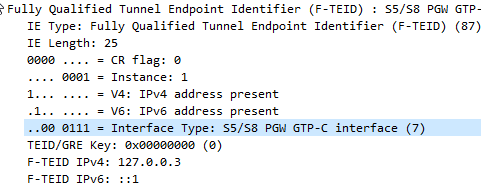# GTPv2 – F-TEID Interface Types

I’ve been working on a ePDG for VoWiFi access to my IMS core.

This has led to a bit of a deep dive into GTP (easy enough) and GTPv2 (Bit harder).

The Fully Qualified Tunnel Endpoint Identifier includes an information element for the Interface Type, identified by a two digit number.

In the end I found the answer in 3GPP TS 29.274, but thought I’d share it here.

I also found how this data is encoded on the wire is a bit strange,

In the example above the Interface Type is 7,

This is encoded in binary which give us 111.

This is then padded to 6 bits to give us 000111.

This is prefixed by two additional bits the first denotes if IPv4 address is present, the second bit is for if IPv6 address is present.

This is then encoded to hex to give us 87

Here’s my Python example;

``````interface_type = int(7)
interface_type = "{0:b}".format(interface_type).zfill(6)   #Produce binary bits
ipv4ipv6 = "10" #IPv4 only
interface_type = ipv4ipv6 + interface_type #concatenate the two
interface_type  = format(int(str(interface_type), 2),"x") #convert to hex``````